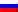# Edmonds algorithm

Contenus

## Edmonds algorithm

The objective of the Edmonds algorithm is to find a perfect coupling (of maximum cardinality) in an assignment problem.

The coupling problem is as follows:

Construct a bipartite graph (the left elements are connected only to the right elements by arcs, the straight elements have no outgoing connection) such that the left elements are the machines and the straight elements are the tasks to be performed. The arcs have capacity 1 and cost according to the assignment table. If a flow passes through an edge (i, j), this amounts to saying that machine i performs task j.
In order to complete the bipartite graph to make it a flow problem, each element on the left is linked as a terminal vertex to a fictitious source. Each element on the line is linked as an original vertex to a fictitious well. All these arcs have capacity 1 and cost 0. In order to solve the allocation problem, we apply a maximum flow algorithm at minimum cost to the constructed bipartite graph.FRFRENRUES